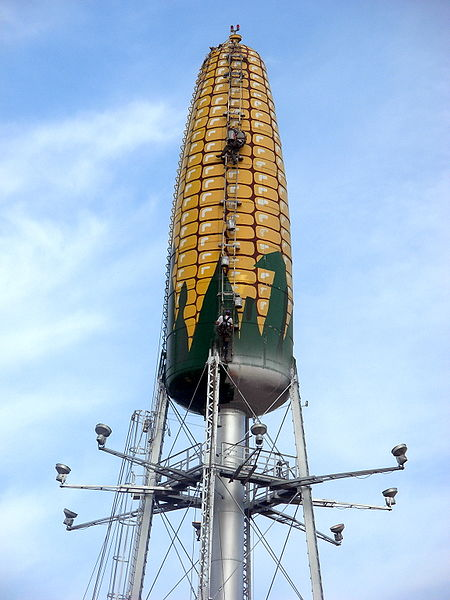# Maximizing The Cylinder's Surface Area

Calculus Level 3Given a cylinder with a fixed volume of 900 $cm^{3}$, is it possible to maximize its TOTAL (not lateral) surface area?

Hint: This problem is easiest to visualize while optimizing by using variable r = radius and h = height.

###### Image credit: [Jonathunder]{http://en.wikipedia.org/wiki/File:CornWaterTower.JPG)
×

Problem Loading...

Note Loading...

Set Loading...